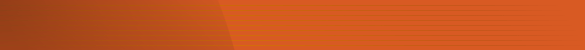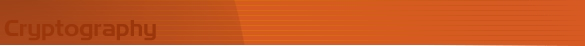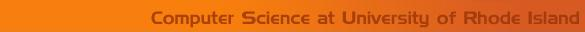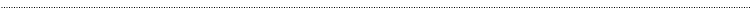Home Page Introduction Classical Ciphers Enigma Machine DES Key Agreement PGP R.S.A. Number Theory Other LinksPublickey CryptographyKid-RSA

Kid-RSA is a public-key cipher system proposed by Neal Koblitz for pedagogic purposes and published in . It is an asymmetric cryptosystem similar to RSA, but is simpler than RSA.

Setup

A person (Alice) chooses four numbers a, b, a1, b1. Then Alice sets

Ma * b -1

ea1 * M + a

db1 * M + b

n = (e * d ) / M = a1 * b1 * M + a * b1 + a1 * b +1

Now Alice Public key (n, e) and her private key is d.

To send Alice a plaintext P, one uses the function  Ce * P ( mod n).

Then Alice can decipher the ciphertext by using the function P = C * d ( mod n).

Note:   The plaint text has to be a number in the range of  0 to n-1. So for this system the plaintext or blocks of plaintext hast to converted into numbers in the range of 0 to n-1.

Encryption and Decryption

To send Alice a plaintext P, one uses the function  Ce * P ( mod n);

Then Alice can decipher the ciphertext by using the function P = C * d ( mod n);

Since Alice publishes e and n, any one who wants to send encrypted messages to Alice can do so, but these messages cannot be decrypted without the knowledge of d. d is kept as secret and only Alice knows it, so only she can decrypt messages.

Example

Let

a = 9, b = 11, a1 = 5, b1 = 8;

There fore

 M (a * b) - 1 (9 * 11) -1 98 e (a1 * M) + a (5 * 98) + 9 499 d (b1 * M) + b (8 * 98) + 11 795 n ((e * d) - 1)/M ((499 * 795) -1) / 98 4048

Let the message be  P = 538.

Encryption

C  =  P * e ( mod n)  = 499 * 538 (mod 4048 ) =  268462 (mod 4048 ) = 1294

Decryption

P = C * d ( mod n)   = 1294 * 795 (mod 4048 ) = 1028730  (mod 4048 )  =538